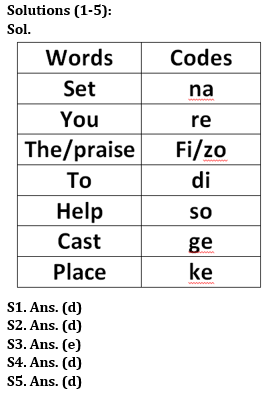Latest Banking jobs   »

# Reasoning Quizzes Quiz For IRDA AM 2023-18th May

Directions (1-5): Study the following information carefully and answer the questions accordingly.
In an artificial language,
‘Set you the praise’ is written as ‘re fi zo na’,
‘Help to set you’ is written as ‘so re na di’,
‘Place cast to set the praise’ is written as ‘ge fi na di ke zo’,
‘Help to cast’ is written as ‘di so ge’.

Q1. Which of the following words are coded as ‘re di so’?
(a) Praise you to
(b) To cast you
(c) Set you to
(e) Can’t be determined

Q2. What will be the code for ‘place set you’?
(a) so di na
(b) ke na di
(c) na di so
(d) ke re na
(e) Cannot be determined

Q3. What will be the code for ‘praise’?
(a) ge
(b) re
(c) zo
(d) fi
(e) Cannot be determined

Q4. Which of the following word is coded as ‘so’?
(a) Cast
(b) To
(c) Set
(d) Help
(e) None is correct

Q5. What will be to code for ‘place’?
(a) di
(b) zo
(c) na
(d) ke
(e) Cannot be determined

Directions (6-10): These questions are based on the following arrangement. Study it

8 H % 3 7 T A 4 # 6 B I @ R 1 Q © L E 2 K \$ U 5 9

Q6. Which element is fifth to the right of fifteenth element from the right end?
(a) Q
(c) L
(d) 1
(e) None of these

Q7. Four of the following five are alike in a certain way based on their positions in the above arrangement and so form a group. Which is the one that does not belong to the group?
(a) 3T7
(b) 6IB
(c) EQ1
(d) 2\$K
(e) U95

Q8. How many such symbols are in the above arrangement each of which is immediately followed by a number but not immediately preceded by another number?
(a) None
(b) One
(c) Two
(d) Three
(e) Four

Q9. If all the symbols are dropped from the above arrangement which element will be ninth from the left end?
(a) B
(b) 6
(c) 4
(d) I
(e) None of these

Q10. What will come in place of the question mark in the following series based on the above arrangement?
7T3 4#A BI6 ?
(a) R@I
(b) IQR
(c) R1@
(e) None of these

SolutionsSolutions (6-10):
S6. Ans.(a)
S7. Ans.(c)
S8. Ans.(b)
Sol. H%3
S9. Ans.(a)
Sol. B is 9th element from left after dropping symbols.
S10. Ans.(c)
Sol. R1@## FAQs

### How many sections are there in the IRDAI exam?

There are 4 sections in the IRDAI assistant manager online preliminary exam. They are Reasoning, English Language, General awareness, Quantitative aptitude.

#### Congratulations!Union Budget 2023-24: Free PDF